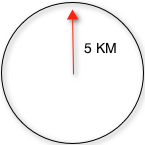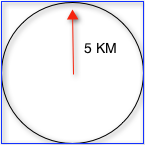# Spatial Search with SolrSpatial Search

Solr supports location data for use in spatial/geospatial searches. Using spatial search, you can:

• Index points or other shapes
• Filter search results by a bounding box or circle or by other shapes
• Sort or boost scoring by distance between points, or relative area between rectangles

Following field types are available for spatial search:

• LatLonType – Better for distance sorting/boosting
• SpatialRecursivePrefixTreeFieldType (RPT for short) – Fast filter performance

RPT offers more features than LatLonType and fast filter performance, although LatLonType is more appropriate when efficient distance sorting/boosting is desired. They can both be used simultaneously for what each does best – LatLonType for sorting/boosting, RPT for filtering.

Indexing:

For indexing geodetic points (latitude and longitude), supply the pair of numbers as a string with a comma separating them in latitude then longitude order. For non-geodetic points, the order is x,y for PointType, and for RPT you must use a space instead of a comma, or use WKT(Well-known text (WKT) is a text markup language for representing vector geometry objects Ex: POLYGON ((30 10, 40 40, 20 40, 10 20, 30 10)).

Searching documents based on their location:

There are 2 types of Spatial filters

1. Geofilt filter 2. Bbox filter

Both filters support following parameters:

 Parameter Description d the radial distance, usually in kilometers. (RPT & BBoxField can set other units via the setting distanceUnits) pt the center point using the format “lat,lon” if latitude & longitude. Otherwise, “x,y” for PointType or “x y” for RPT field types. sfield a spatial indexed field score (Advanced option; RPT and BBoxField field types only) If the query is used in a scoring context (e.g. as the main query in q), this local-param determines what scores will be produced. Valid values are: none – A fixed score of 1.0. (the default) kilometers – distance in kilometers between the field value and the specified center point miles – distance in miles between the field value and the specified center point degrees – distance in degrees between the field value and the specified center point distance – distance between the field value and the specified center point in the distanceUnits configured for this field recipDistance – 1 / the distance When used with BBoxField,additional options are supported: overlapRatio – The relative overlap between the indexed shape & query shape. area – haversine based area of the overlapping shapes expressed in terms of the distanceUnits configured for this field area2D – cartesian coordinates based area of the overlapping shapes expressed in terms of the distanceUnits configured for this field filter (Advanced option; RPT and BBoxField field types only) If you only want the query to score (with the above score local-param), not filter, then set this local-param to false.

geofilt:

The geofilt filter allows you to retrieve results based on the geospatial distance (the “great circle distance”) from a given point. Another way of looking at it is that it creates a circular shape filter. For example, to find all documents within five kilometers of a given lat/lon point, you could enter &q=*:*&fq={!geofilt sfield=store}&pt=45.15,-93.85&d=5. This filter returns all results within a circle of the given radius around the initial point:bbox:

The bbox filter is very similar to geofilt except it uses the bounding box of the calculated circle. See the blue box in the diagram below. It takes the same parameters as geofilt. Here’s a sample query: &q=*:*&fq={!bbox sfield=store}&pt=45.15,-93.85&d=5. The rectangular shape is faster to compute and so it’s sometimes used as an alternative to geofilt when it’s acceptable to return points outside of the radius. However, if the ideal goal is a circle but you want it to run faster, then instead consider using the RPT field and try a large “distErrPct” value like 0.1 (10% radius). This will return results outside the radius but it will do so somewhat uniformly around the shape.

The distance-error-percent of a query shape in Lucene spatial is, in a nutshell, the percent of the shape’s area that is an error epsilon when considering search detail at its edges. The default is 2.5%, for reference. However, as configured, it is read in as a fraction:

<fieldType name=”location_2d_trie” class=”solr.SpatialRecursivePrefixTreeFieldType” distErrPct=”0.025? maxDetailDist=”0.001? />Filtering by an arbitrary rectangle

Sometimes the spatial search requirement calls for finding everything in a rectangular area, such as the area covered by a map the user is looking at. For this case, geofilt and bbox won’t cut it. This is somewhat of a trick, but you can use Solr’s range query syntax for this by supplying the lower-left corner as the start of the range and the upper-right corner as the end of the range. Here’s an example: &q=*:*&fq=store:[45,-94 TO 46,-93]. LatLonType does not support rectangles that cross the dateline, but RPT does. If you are using RPT with non-geospatial coordinates (geo=”false”) then you must quote the points due to the space, e.g. “x y”.

Optimization: Solr Post Filtering

Most likely, the fastest spatial filters will be to simply use the RPT field type. However, sometimes it may be faster to use LatLonType with Solr post filtering in circumstances when both the spatial query isn’t worth caching and there aren’t many matching documents that match the non-spatial filters (e.g. keyword queries and other filters). To use Solr post filtering with LatLonType, use the bbox or geofilt query parsers in a filter query but specify cache=false and cost=100 (or greater) as local-params. Here’s a short example:

&q=…mykeywords…&fq=…someotherfilters…&fq={!geofilt cache=false cost=100}&sfield=store&pt=45.15,-93.85&d=5

Distance Function Queries

There are four distance function queries: geodist, see below, usually the most appropriate; dist, to calculate the p-norm distance between multi-dimensional vectors; hsin, to calculate the distance between two points on a sphere; and sqedist, to calculate the squared Euclidean distance between two points.

In this article we will see how to use geodist function query :

geodist

geodist is a distance function that takes three optional parameters: (sfield,latitude,longitude).

geodist is a function query that yields the calculated distance. This gives the flexibility to do a number of interesting things, such as sorting by the distance (Solr can sort by any function query), or combining the distance with the relevancy score, such as boosting by the inverse of the distance. You can use the geodist function to sort results by distance or score return results.

For example, to sort your results by ascending distance, enter …&q=*:*&fq={!geofilt}&sfield=store&pt=45.15,-93.85&d=50&sort=geodist() asc.

Or you could use the distance function as the main query (or part of it) to get the distance as the document score:

…&q={!func}geodist()&sfield=store&pt=45.15,-93.85&sort=score+asc.

More Examples

Here are a few more useful examples of what you can do with spatial search in Solr.

Use as a Sub-Query to Expand Search Results

Here we will query for results in Mumbai, Maharashtra, or within 50 kilometers of 28.6,77.2 (near Delhi):

&q=*:*&fq=(state:”MH” AND city:”Mumbai”) OR {!geofilt}&sfield=store&pt=28.6,77.2 &d=50&sort=geodist()+asc

Facet by Distance

To facet by distance, you can use the Frange query parser:

&q=*:*&sfield=store&pt=45.15,-93.85&facet=true&facet.query={!frange l=0 u=5}geodist()&facet.query={!frange l=5.001 u=3000}geodist()

There are other ways to do it too, like using a {!geofilt} in each facet.query.

&q=*:*&sfield=store&pt=45.15,-93.85&facet=true&facet.query={!geofilt d=10 key=d10}&facet.query={!geofilt d=20 key=d20}&facet.query={!geofilt d=50 key=d50007D

Boost Nearest Results

Using the DisMax or Extended DisMax, you can combine spatial search with the boost function to boost the nearest results:

&q.alt=*:*&fq={!geofilt}&sfield=store&pt=45.15,-93.85&d=50&bf=recip(geodist(),2,200,20)&sort=score desc